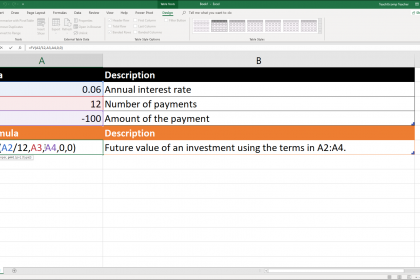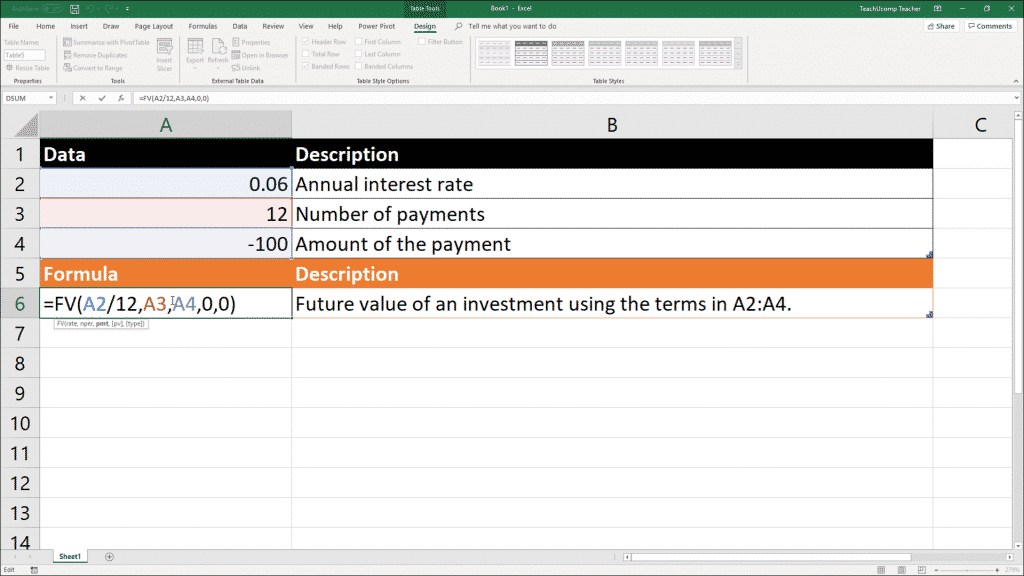# The Fv Function- Excel for Lawyers Tutorial

/ / Excel 2016, Excel 2019, Excel for Office 365, Latest, Microsoft, Microsoft Excel Training for Lawyers, Office 2016, Office 2019, Office 365## The Fv Function: Overview

The Fv function assists you in determining the future value of an investment based on periodic but constant payments with a constant interest rate. The future value is the total amount that a series of payments will be worth at a specified future time. For example, if you saved \$100 a month over one year into an account that earns 6% interest, the future value in a year would be \$1233.56.###### The Fv Function- Excel for Lawyers Tutorial: A picture of a user writing the Fv function in Excel.

This function can be useful in determining the future value within an interest-bearing account, such as an IOLTA account, for example. Let’s examine the syntax of the Fv function and the arguments that the function uses.

 Formula Syntax: FV(rate, nper, pmt, [pv], [type]) Arguments: Rate Required The interest rate per period. For example, the monthly interest rate on an account that pays 6% interest per year would be 6%/12, or .5%. You could enter 6%/12, .5%, or 0.005 into the formula as the rate argument. Nper Required The total number of periods. For example, if you placed \$100 into an account each month for a year, the nper argument would be 12. Pmt Required The unchanging payment made each period. Typically includes payment and interest, but no fees. For example, if you placed \$100 into an account each month for a year, the pmt argument would be -100. Pv Optional The present value or lump-sum amount that a series of future payments is worth right now. If omitted, this argument is assumed to be zero. Note that if the pv argument is omitted, then the pmt argument is required. Type Optional Enter 0, or omit, if payments are made at the end of the period. Enter 1 if the payments are made at the beginning of each period.

## The Fv Function: Instructions

1. Select the cell in the worksheet where you want to display the result of the function.
2. Type =FV(rate, nper, pmt, [pv], [type]), where rate, nper, pmt, pv and type are the values, or cell references to cells within the worksheet that contain the values, that you wish to use. Note that the first three are required arguments and the last two are optional.
3. Exit the cell to see the result of the function.

## The Fv Function: Video Lesson

The following video lesson, titled “The Fv Function,” shows how to use the Fv function in Excel 2019 and 365. This video lesson is from our complete Excel for Lawyers training, titled “Mastering Excel Made Easy for Lawyers v.2019 and 365.”

TOP
\$199 \$49 Entire Library! -1 Days 23 Hours 3 Minutes 42 Seconds      Get a better deal!